# UFR 4-06 Evaluation

(diff) ← Older revision | Latest revision (diff) | Newer revision → (diff)

# Evaluation

## Comparison of CFD calculations with Experiments

The experimental results of Clausen et al  are included on the ERCOFTAC database for each measurement station identified in section 4. Armfield et al  presented a comparison of the CFD results with the experimental data including radial variation of axial velocity, radial variation of swirl velocity, radial variation of turbulent kinetic energy and radial variation of Reynolds stresses. Examples of these comparisons are shown in Figures 5, 6, 7, 8 and 9. Measurement stations 3, 5 and 7 are located 100mm, 250mm and 405mm downstream of the diffuser inlet respectively (see Figure 4).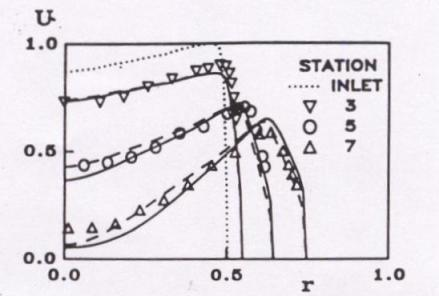Figure 5 Radial Variation of Normalised Axial Velocity
() ASM, (----) k-ε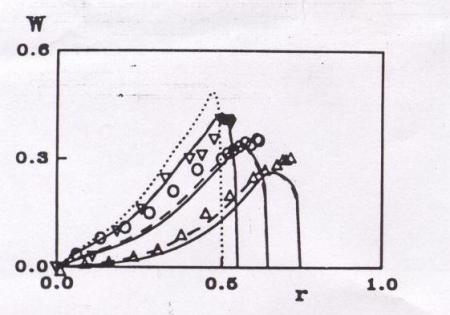Figure 6 Radial Variation of Normalised Swirl Velocity
() ASM, (----) k-ε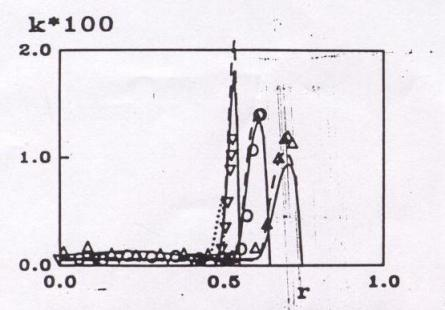Figure 7 Radial Variation of Normalised Turbulent Kinetic Energy
() ASM, (----) k-ε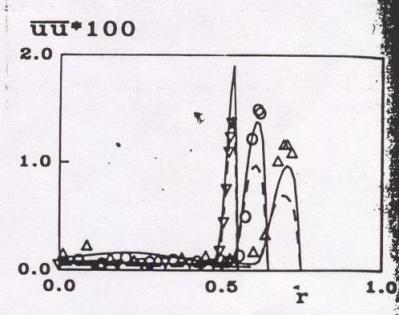Figure 8 Radial Variation of Normalised Reynolds Stress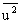() ASM, (----) k-ε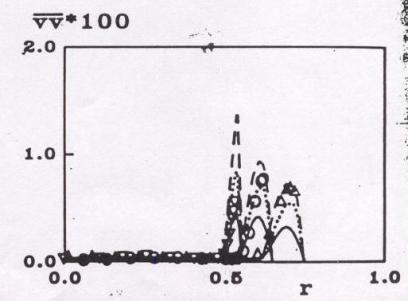Figure 9 Radial Variation of Normalised Reynolds Stress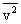() ASM, (----) k-ε (the dotted line is the ASM without wall echo terms) 

Based on the findings of Armfield et al , overall, good prediction of the mean and turbulence quantities was obtained using both turbulence models with a two layer wall function based approach. However, the ASM gave slightly better overall predictions of the turbulence quantities. The use of a two layer, rather than a single layer, wall function was found to be necessary to predict accurately the level, location and the axial variation of the near wall peak in turbulence quantities. These findings have been further supported by the work of Armfield and Fletcher , Cho and Fletcher  and Page et al .

The summary report of EDF  discussed the results of eleven simulations of the test case flow, as described in section 5, and the findings are reproduced below.

• In some of the calculations, the measured k-distribution at the inflow boundary was not used as the initial profile in the boundary layer, but different (mainly lower) distributions were used. The initial k-level in the k-ω model calculation was far too high, causing excessive k and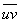levels also in the initial diffuser region. The low Re k-ε model calculations also develop excessive levels of k and of the shear stressesand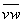, which persist throughout the whole diffuser. In particular, the large value ofnear the wall is strange and entirely unrealistic. Also, the swirl velocity in these calculations is somewhat higher than in the experiments at most stations, indicating that the swirl number was not exactly matched to the experiments. The ASM and Reynolds stress model calculations of k and shear stresses are also initially all too high. The ASM and the RSM calculations from TH Aachen [see reference ] stay high all the way through the diffuser while the RSM calculations of EC Lyon [see reference ] fall below the experimental levels towards the outlet of the diffuser. There are hence considerable differences in the calculated turbulence quantities between the calculations using nominally the same LRR Reynolds stress model. The best argument concerning the turbulence quantities was obtained by U. Brussels [see reference ] with the standard k-ε model and two non-linear k-ε model versions. The Hirsch-Khodak version produces the better results and is in fact the only model that can simulate reasonably well the distribution of the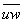stress. For the other turbulence quantities the standard k-ε model gives results equally close to the measurements.

• The reduction in U velocity in the center portion towards the outlet of the diffuser is under-predicted by most models. Only the low Re k-ε model calculation produces nearly the correct reduction. This may have to do with the fact that, compared with the other calculations, this model yields higher swirl velocity and shear stresses which are, however, also higher than the experimentally observed values. The LRR calculation from Lyon [see reference ] also yields lower U-velocity in the center portion in better agreement with the measurements, but strangely this is associated with smaller shear stresses (significantly below the experimental levels) than in the other models. With these two exceptions, there is generally good agreement among the various predictions about the development of the U and W velocity profiles; only at the station closest to the outlet there is considerable spread, the k-ε model yielding too high U velocity at the axis. The streamwise distribution of pressure in the diffuser is calculated well by all models. The various k-ε model calculations also produced reasonable distributions of the axial and circumferential wall shear stress, while some of the other models yield too high values.

• Altogether it seems that the standard k-ε model with wall functions gives results in this case which, apart from an insufficient reduction in the center line velocity, are reasonable for all quantities that are of practical interest. More complex models do not seem to yield a marked improvement. It appears that the swirl is not strong enough in this case to show the superiority of Reynolds stress models reported in the literature for swirling flows.